10 天拿到字节跳动 Java 岗位 offer，Java 编程入门自学4 步套路，解决动态规划问题

1、确定问题状态

• 提炼最后一步

• 的问题转化

2、转移方程，把问题方程化 3、按照实际逻辑设置初始条件和边界情况 4、确定计算顺序并求解

**正常人第一反应思路:**最少硬币组合?优先使用大面值硬币——7+7+7+5=26 额？可求解目标是 27 啊……改算法——7+7+7+2+2+2=27，总共用了 6 枚硬币正好 27 元.实际正确答案：7+5+5+5+5=27，才用了 5 枚硬币。所以这里贪心算法是不正确的。

第一步，确定问题状态。

A、确定状态首先提取【最后一步】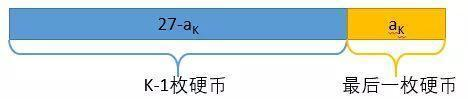B、**转化子问题。**最后一步 aK 提出来之后，我们只要求出“最少用多少枚硬币可以拼出 27- aK”就可以了。

f(27) = min{f(27-2)+1, f(27-5)+1, f(27-7)+1}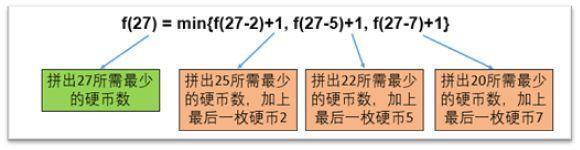第二步，转移方程，把问题方程化。

f[X] = min{f[X-2]+1, f[X-5]+1, f[X-7]+1}（动态规划都是要开数组，所以这里改用方括号表示）

// f(X)返回最少用多少枚硬币拼出Xint f(int X) {// 0元钱只要0枚硬币if (X == 0) return 0;// 初始化用无穷大（为什么是正无穷？）int res = MAX_VALUE;// 最后一枚硬币是2元if (X >= 2) {res = Math.min(f(X – 2) + 1, res);}// 最后一枚硬币是5元if (X >= 5) {res = Math.min(f(X – 5) + 1, res);}// 最后一枚硬币是7元if (X >= 7) {res = Math.min(f(X – 7) + 1, res);}return res;}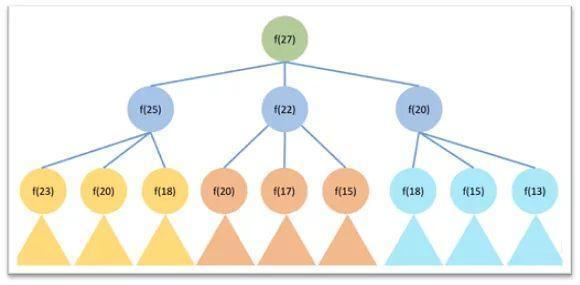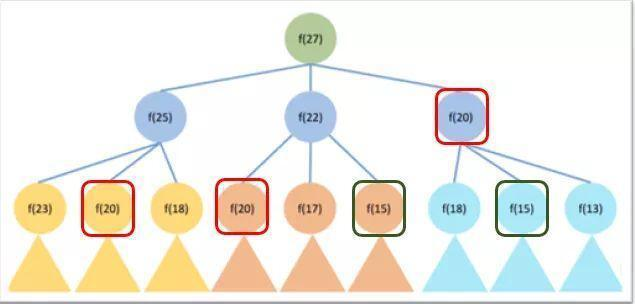第三步，按照实际逻辑设置边界情况和初始条件。

**【必做】**否则即使转移方程正确也大概率无法跑通代码。

f[X] = min{f[X-2]+1, f[X-5]+1, f[X-7]+1}的边界情况是[x-2]/[x-5]/[x-7]不能小于 0（硬币面值为正），也不能高于 27。

**特殊情况：**本题的 F对应的情况为 F[-2]、F[-5]、F[-7]，按照上文的边界情况设定结果是正无穷。

第四步，确定计算顺序并计算求解

**原题练习及实际代码：**这道题是 lintcode 编号 669 的 Coin Change 问题。代码如下：

public int coinChange(int[] A, int M){// A = [2,5,7]// M = 27int[] f = new int[M + 1];int n = A.length; // 硬币的种类// 初始化, 0个硬币f = 0;// f, f, ... , f = Integer.MAX_VALUEfor (int i = 1; i <= M; i++){f[i] = Integer.MAX_VALUE;}for (int i = 1; i <= M; i++){// 使用第j个硬币 A[j]// f[i] = min{f[i-A]+1, ... , f[i-A[n-1]]+1}for (int j = 0; j < n; ++j){// 如果通过放这个硬币能够达到重量iif (i >= A[j] && f[i - A[j]] != Integer.MAX_VALUE) {// 获得i的重量的硬币数就可能是获得i-A[j]重量硬币数的方案+1// 拿这个方案数量与原本的方案数打擂台，取最小值就行f[i] = Math.min(f[i - A[j]] + 1, f[i]);}}}if (f[M] == Integer.MAX_VALUE){return -1;}return f[M];}

1、这是求最值问题，用动态规划方式求解。2、进入求解过程，先确定问题状态

• 提炼最后一步（最优策略中使用的最后一枚硬币 aK）-子问题转化 （最少的硬币拼出更小的面值 27-aK）3、构建转移方程 f[X] = min{f[X-2]+1, f[X-5]+1, f[X-7]+1}（求 min 是因为题目要求求最小）4、设置初始条件和边界情况 f = 0, 如果不能拼出 Y，f[Y]=正无穷 5、确定计算顺序并计算求解 f, f, f……

总结66 个 Java 面试知识点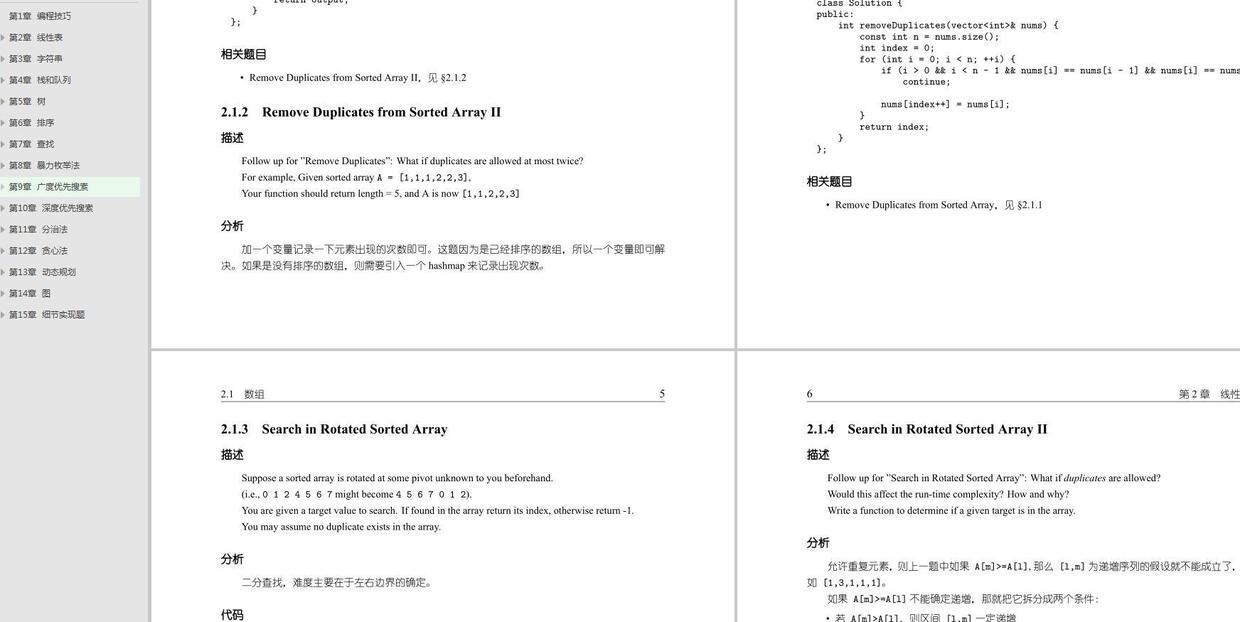程序猿一枚

VX：Lzzzzzz63 领取资料 2021.07.29 加入

评论